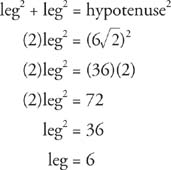## Special Right Triangles

Central angles are probably the angles most often associated with a circle, but by no means are they the only ones. Angles may be inscribed in the circumference of the circle or formed by intersecting chords and other lines.

Isosceles right triangle. An isosceles right triangle has the characteristic of both the isosceles and the right triangles. It has two equal sides, two equal angles, and one right angle. (The right angle cannot be one of the equal angles or the sum of the angles would exceed 180°.) Therefore, in Figure 1 , Δ ABC is an isosceles right triangle, and the following must always be true.Figure 1 An isosceles right triangle.Figure 2 The ratios of the sides of an isosceles right triangle

Example 1: If one of the equal sides of an isosceles right triangle is 3, what are the measures of the other two sides?

Method 1: Using the ratio x : x : xfor isosceles right triangles, then x = 3, and the other sides must be 3 and 3.

Method 2. Using the Pythagorean Theorem and the fact that the legs of this right triangle are equal,The two sides have measures of 3 and 3Example 2: If the diagonal of a square is 6, find the length of each of its sides.Figure 3 A diagonal of a square helps create two congruent isosceles right triangles.

The ratio x : x : xfor isosceles right triangles can now be applied where x= 6. So x = 6, and each side of the square has a measure of 6.

Method 2: Use the Pythagorean Theorem. 6represents the hypotenuse.Figure 4 Finding the unknown parts of this right triangle

Therefore, each side of the square has a measure of 6.

45° + 90° + x° = 180° (The sum of the angles of a triangle = 180°.) x = 45°

Therefore, this is an isosceles right triangle with the ratio of sides x : x : xBecause one leg is 10, the other must also be 10, and the hypotenuse is 10, soy = 10 and z = 10.

30°− 60°− 90° right triangle. A 30°− 60°− 90° right triangle has a unique ratio of its sides. The ratio of the sides of a 30°−60°−90° right triangle is 1 :: 2 or x : x: 2 x placed as follows.Figure 5 The ratios of the sides of a 30°−60°−90° triangle

Example 4: If the shortest side of a 30°−60°−90° right triangle is 4, what is the measure of the other two sides?

In Figure 6 , x is opposite the 30°. The other two sides are then x(opposite the 60°) and 2 x (opposite the 90°). Because the shortest side is 4, x = 4. Consequently, the other two sides must be 4A and 2(4), or 8.

Example 4: If the shortest side of a 30°−60°−90° right triangle is 4, what is the measure of the other two sides?

In Figure 6 , x is opposite the 30°. The other two sides are then x(opposite the 60°) and 2 x (opposite the 90°). Because the shortest side is 4, x = 4. Consequently, the other two sides must be 4A and 2(4), or 8.Figure 6 Using the shortest side of a 30°−60°−90° triangle to find the remaining sides.

Example 5: If the longer leg of a 30°−60°−90° right triangle is 8, find the length of the hypotenuse.

In Figure 7 , the shorter leg, x, is opposite the 30°. xis the longer leg, and it is opposite the 60°. The hypotenuse is 2 x. Because x= 8x = 8. Because x = 8, then 2 x = 16. The hypotenuse is 16.Figure 7 Using the longer leg of a 30°−60°−90° triangle to find the hypotenuse.

Example 6: Find the length of an altitude in an equilateral triangle with a perimeter of 60 inches.

Figure 8 is an equilateral triangle. Each angle has a measure of 60°. If an altitude is drawn, it creates two 30°−60°−90° right triangles. Because the perimeter is 60 inches, and the three sides are equal in measure, then each side is 20 inches (60 ÷ 3 = 20). The ratio of sides in a 30°−60°−90° right triangle is xx: 2 x. In this problem, the length 20 inches represents the longest side in the 30°−60°−90° right triangle, so 2 x = 20, or x = 10. Because the altitude is the longer leg of the 30°−60°−90° right triangle and its measure is x, the altitude is 10inches long.Figure 8 the perimeter of an equilateral triangle to find an altitude

Back to Top
A18ACD436D5A3997E3DA2573E3FD792A